## In probability and statistics, The Binomial Distribution is a frequency distribution of the possible number of successful outcomes in a given number of trials in each of which there is the same probability of success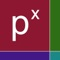# Binomial Distribution

by Donald Schaefer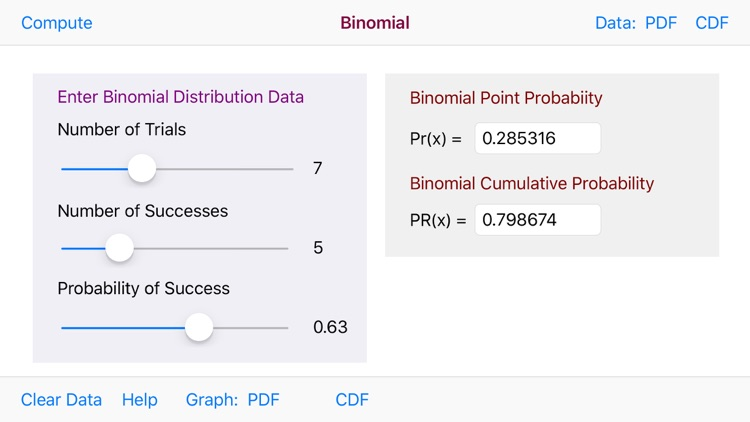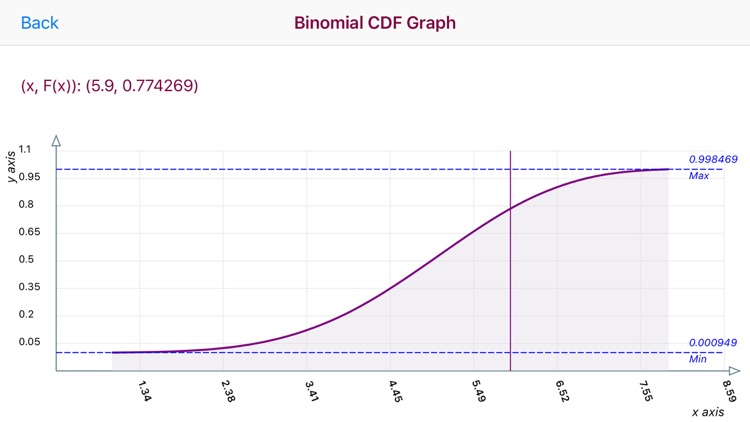Binomial Distribution Offers Apple Watch App
Check the details on WatchAware

In probability and statistics, The Binomial Distribution is a frequency distribution of the possible number of successful outcomes in a given number of trials in each of which there is the same probability of success.### App Details

Version
1.6
Rating
(4)
Size
1Mb
Genre
Education Productivity
Last updated
September 30, 2020
Release date
May 21, 2015

### App Screenshots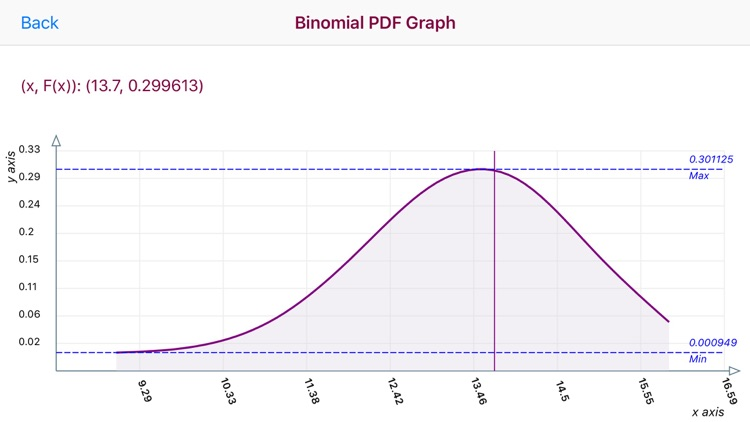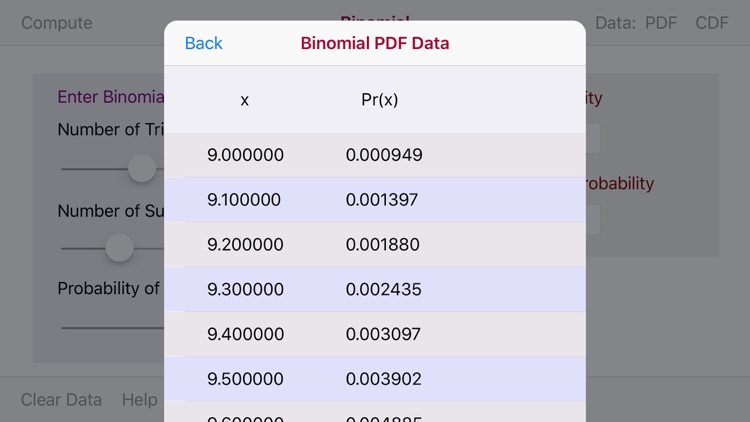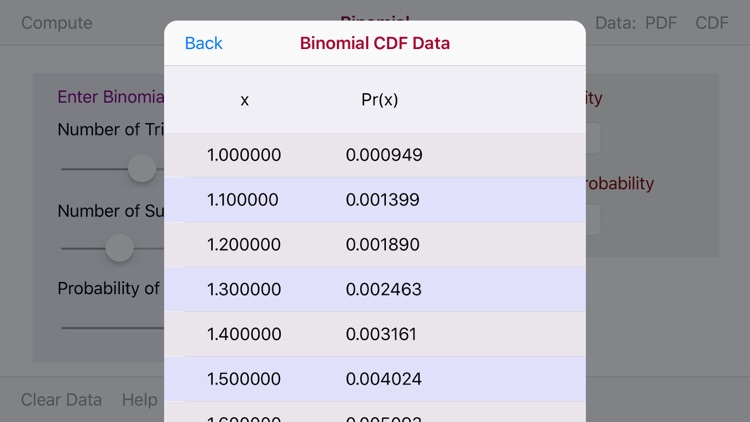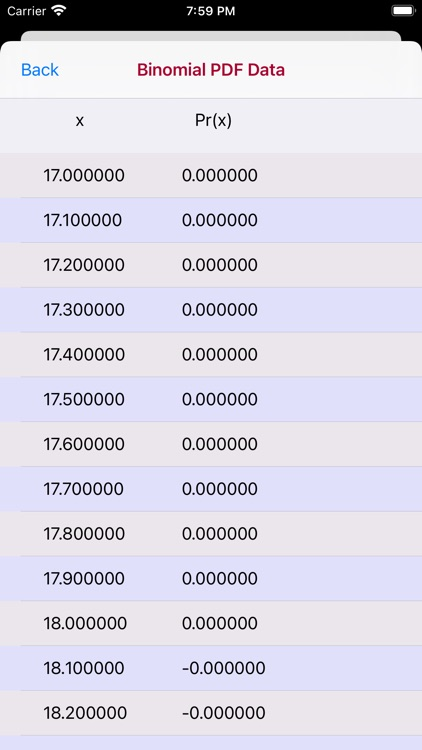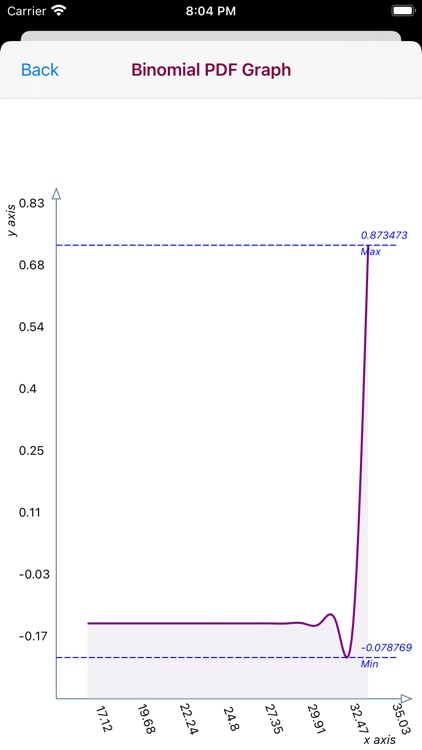### App Store Description

In probability and statistics, The Binomial Distribution is a frequency distribution of the possible number of successful outcomes in a given number of trials in each of which there is the same probability of success.

The Binomial Distribution summarizes the likelihood that a value will take one of two independent values under a given set of parameters or assumptions. The underlying assumptions of the binomial distribution are that there is only one outcome for each trial, that each trial has the same probability of success and that each trial is mutually exclusive.

For Data Entry the Binomial Distribution app for iPad and iPhone utilizes three Sliders to enter the Number of Trials, Number of Success and the Probability of Success variables. The range of the Number of Trials and Number of Successes is [1-20]. The range of the Probability of Success variable is [1.0-100.0] and is divided into 100 1.0 segments. After moving the Sliders to your selected values enter the Compute button.

The Binomial Distribution app graphs the Binomial Proability Density Function f(x) graph utilized the Normal Distribution Approximation to the Binomial Distribution curve. A Data Chart for the PDF values also exists.

Disclaimer:
AppAdvice does not own this application and only provides images and links contained in the iTunes Search API, to help our users find the best apps to download. If you are the developer of this app and would like your information removed, please send a request to [email protected] and your information will be removed.